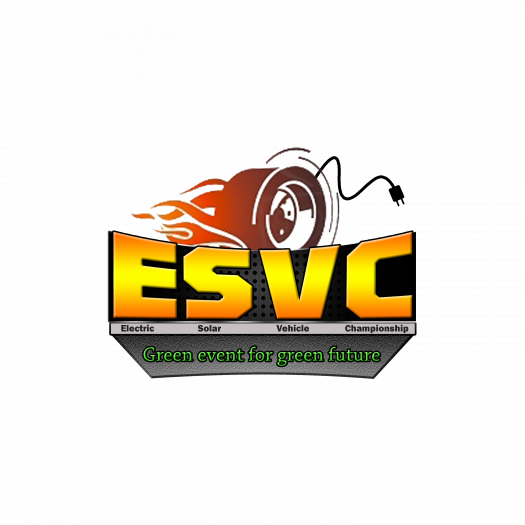# Esvc Season 7.0 Rulebook Test

25 Questions | Total Attempts: 44SettingsCreate your own Quiz.

• 1.
Which one is the correct dimensions for Adventure class?(L*B*H)
• A.

100*50*50 inches

• B.

2540*1522*1522 mm

• C.

2540*1524*1522 mm

• D.

100*60*60 inches

• 2.
The fully-laden solar car must have a minimum ground clearance of?
• A.

5 inches

• B.

6 inches

• C.

4 inches

• D.

8 inches

• 3.
Max. Weight For Adventure class,without the driver.
• A.

200 kg

• B.

180 kg

• C.

150 kg

• D.

160 kg

• 4.
What is the maximum allowable steering system free play.?
• A.

10 degree

• B.

7 degree

• C.

6 degree

• D.

15 degree

• 5.
Min. Wheel diameter Should be ?
• A.

12 inches

• B.

14 inches

• C.

10 inches

• D.

16 inches

• 6.
Min. and Max. tread width of the tyre must be?
• A.

90 mm , 165.1 mm

• B.

98 mm , 165.1 mm

• C.

90 mm , 164.1 mm

• D.

None

• 7.
Min. Required Wall thickness of tube mentioned in rulebook  is?
• A.

1 mm

• B.

2 mm

• C.

3 mm

• D.

0.25 mm

• 8.
Which Type of shape is allowed in three wheel vehicle?
• A.

Delta shape

• B.

• C.

Both

• D.

None

• 9.
The Front Hoop must not be more than _________forward of the steering wheel
• A.

10 inches

• B.

9.6 inches

• C.

9.4 inches

• D.

9.8 inches

• 10.
The distance from the hip point to the top of the head restrain must be
• A.

900 mm

• B.

850 mm

• C.

800 mm

• D.

None of the above

• 11.
Driver must have the total vision range of___as mentioned in rulebook
• A.

150º

• B.

100º

• C.

200º

• D.

None of the above

• 12.
When viewed from front view ,if wheels are tilted outward from vertical axis, then it is called.
• A.

Positive camber

• B.

Negative camber

• C.

Positive caster

• D.

Negative caster

• 13.
The power actually developed inside the engine cylinder is called as
• A.

Indicated power

• B.

Brake power

• C.

Frictional power

• D.

None of these

• 14.
Suspension used must have the total travel of_____as mentioned in rulebook.
• A.

4 inches

• B.

5 inches

• C.

6 inches

• D.

None

• 15.
A cycle consisting of one constant pressure, one constant volume and two isentropic processes is known as
• A.

Carnot cycle

• B.

Stirling cycle

• C.

Otto cycle

• D.

Diesel cycle

• 16.
In Adventure class, teams are allowed to place panels on the roof or over the head of the driver?
• A.

True

• B.

False

• 17.
Maximum Normal Voltage of Accumulator for Adventure class allowed is
• A.

60 V and 40 MJ.

• B.

40 V and 20 MJ.

• C.

60 V and 20 MJ.

• D.

None

• 18.
Allowed Traction Motor shall be a BLDC motor with Peak Power not more than
• A.

2.5 kW.

• B.

4.5 kW.

• C.

1 kW.

• D.

5 kW.

• 19.
Total how many kill switches must be in the vehicle?
• A.

2

• B.

3

• C.

1

• D.

None

• 20.
How many and which Class of Fire extinguishers must be used as mentioned in rulebook ?
• A.

3,A-B

• B.

2,A-B-C

• C.

1,B-C

• D.

2,B-C-D

• 21.
The gap between restraint and the back of the driver’s helmet must be
• A.

30 mm

• B.

5 mm

• C.

15 mm

• D.

25 mm

• 22.
Brake Over travel switch must be wired in___________ as mentioned in rulebook.
• A.

Series

• B.

Parallel

• C.

Both

• D.

None

• 23.
Dimensions of jack point must be
• A.

12*2*0.1968 inches

• B.

12*2*2 inches

• C.

12*3*2 inches

• D.

Any

• 24.
In the case of steel material used in Frame, minimum carbon percentage should be
• A.

0.20%

• B.

0.50%

• C.

0.10%

• D.

1%

• 25.
At how much volt the accessories(lights,horn,indicators) are Operated in car
• A.

12 V DC

• B.

12 V AC

• C.

24 V DC

• D.

24  V AC

Related TopicsBack to top
×

Wait!
Here's an interesting quiz for you.